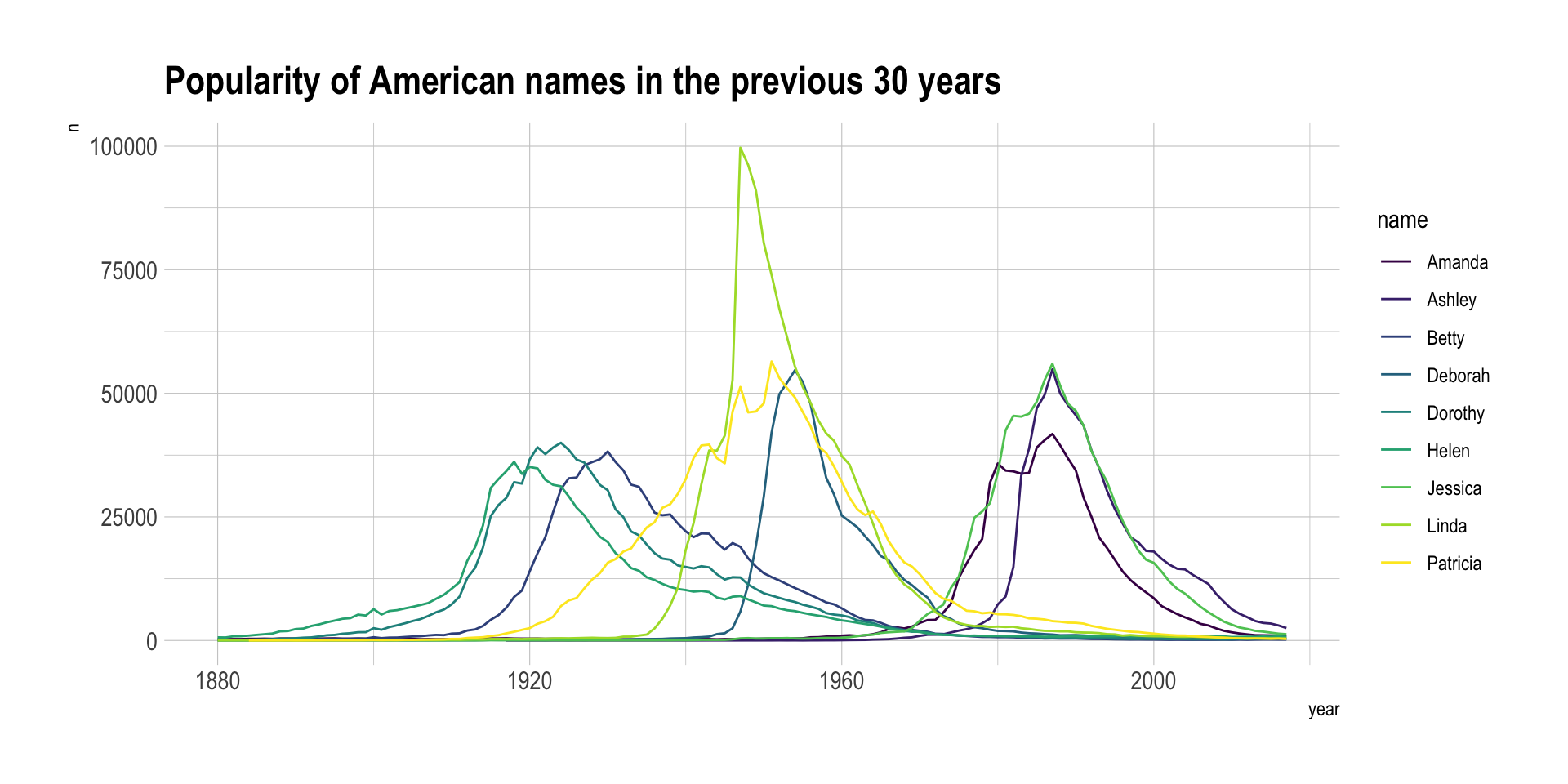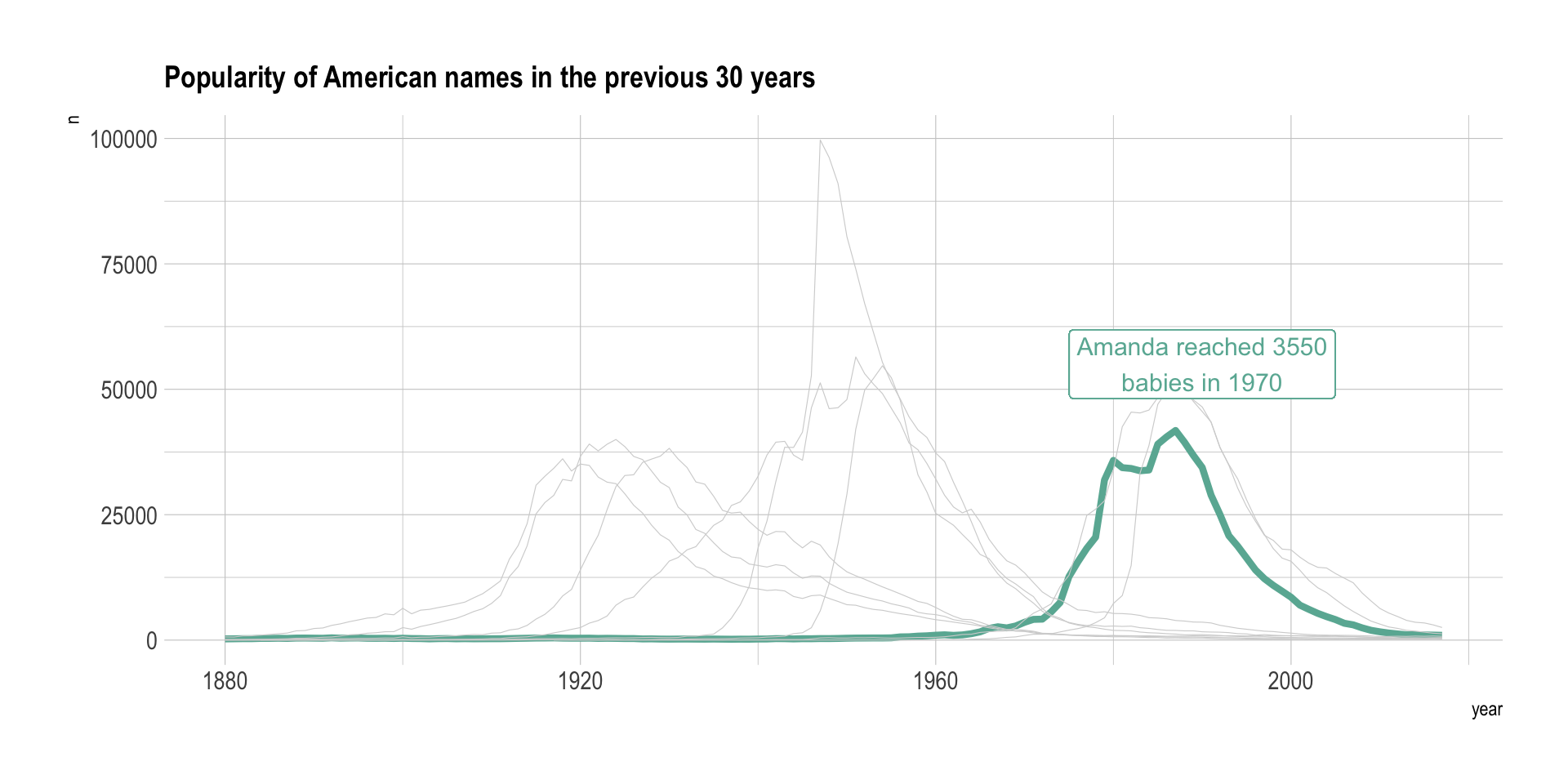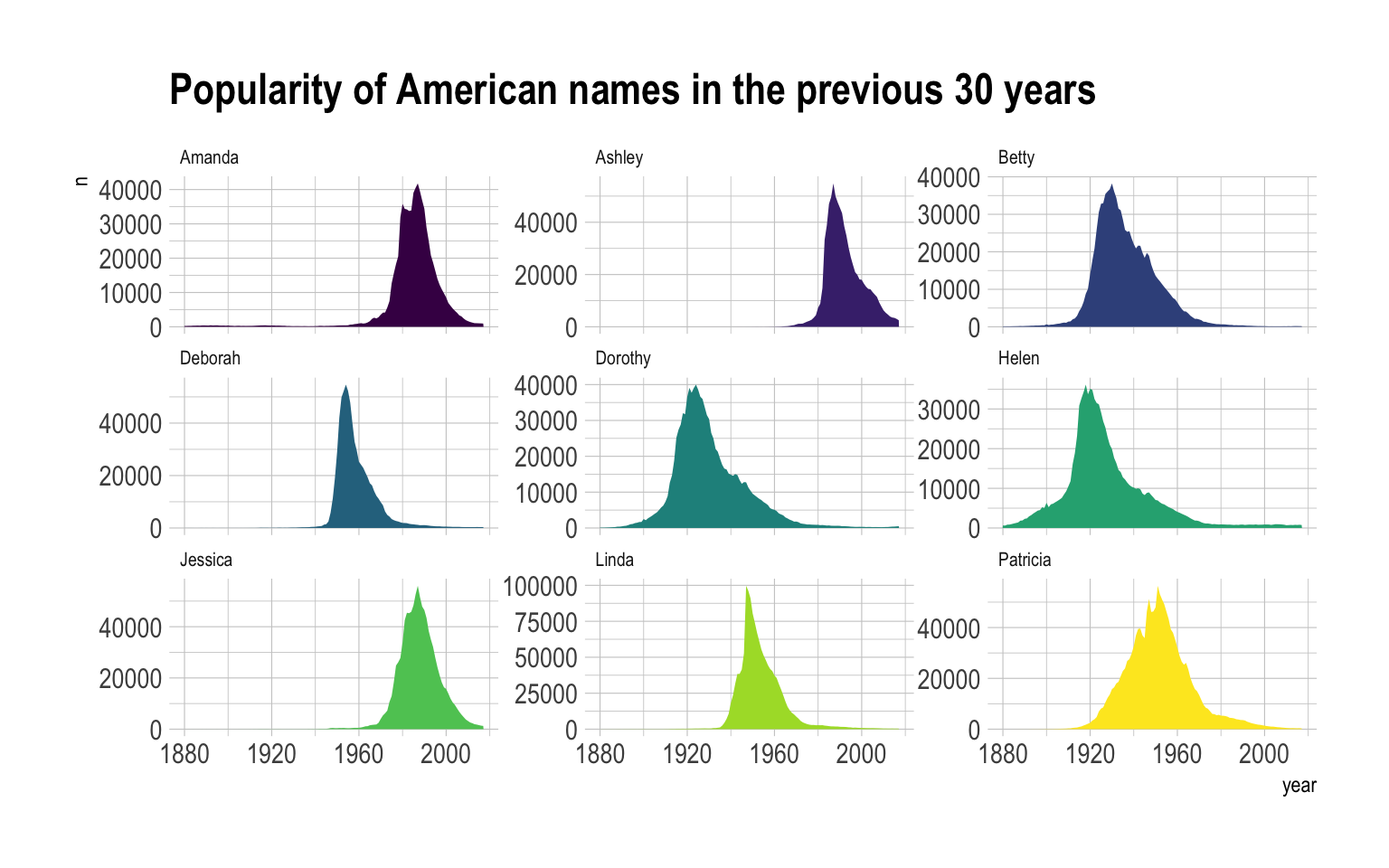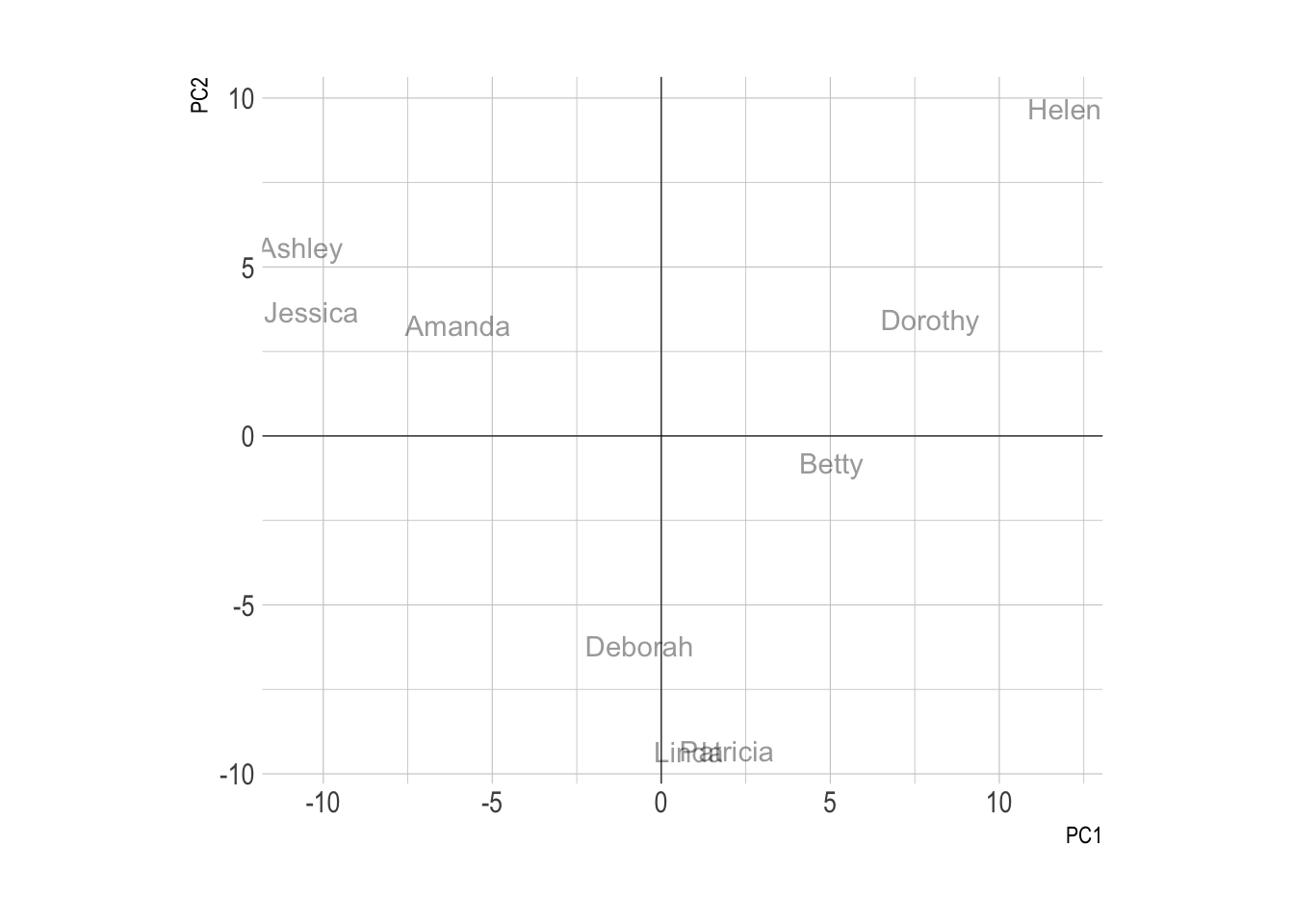This document aims to describe a few dataviz that can be applied to a dataset containing an ordered numeric variable, a categoric variable and another numeric variable. An an example we will consider the evolution of baby name frequencies in the US between 1880 and 2015. This dataset is available through the babynames R library and a `.csv` version is available on github. It looks as follow:

``````# Libraries
library(tidyverse)
library(hrbrthemes)
library(kableExtra)
options(knitr.table.format = "html")
library(babynames)
library(streamgraph)
library(viridis)
library(DT)
library(plotly)

data <- babynames %>%
filter(name %in% c("Ashley", "Amanda", "Jessica",    "Patricia", "Linda", "Deborah",   "Dorothy", "Betty", "Helen")) %>%
filter(sex=="F")

# Show long format
data %>%
select(year, name, n) %>%
arrange(name) %>%
kable() %>%
kable_styling(bootstrap_options = "striped", full_width = F)``````
year name n
1880 Amanda 241
1880 Betty 117
1880 Dorothy 112
1880 Helen 636
1880 Linda 27

# A note on data format

It is of importance to note that the following table provides exactly the same information, but in a different format. We call it ‘long’ and ‘wide’ format and most tools provide function to go from one to the other. In any case, we will apply the same kind of visualization to both format.

``````wide <- data %>%
select(year, name, n) %>%

wide %>%
select(1:5) %>%
kable() %>%
kable_styling(bootstrap_options = "striped", full_width = F)``````
year Amanda Ashley Betty Deborah
1880 241 NA 117 12
1881 263 NA 112 14
1882 288 NA 123 15

# Line plot

The first obvious solution to represent this dataset is to produce a line chart. Each baby name is represented by a line. The X axis gives the year and the Y axis shows the number of babies.

``````data %>%
ggplot( aes(x=year, y=n, group=name, color=name)) +
geom_line() +
scale_color_viridis(discrete = TRUE) +
theme(legend.position="none") +
ggtitle("Popularity of American names in the previous 30 years") +
theme_ipsum()``````Line charts tend to be too cluttered as soon as more than a few groups are displayed. This is a common mistake in dataviz, so common that it has been named spaghetti chart. Thus this solution is usually applied if you want to highlight a specific group from the whole dataset. For example, let’s highlight the evolution of Amanda compared to the other first names:

``````data %>%
mutate( highlight=ifelse(name=="Amanda", "Amanda", "Other")) %>%
ggplot( aes(x=year, y=n, group=name, color=highlight, size=highlight)) +
geom_line() +
scale_color_manual(values = c("#69b3a2", "lightgrey")) +
scale_size_manual(values=c(1.5,0.2)) +
theme(legend.position="none") +
ggtitle("Popularity of American names in the previous 30 years") +
theme_ipsum() +
geom_label( x=1990, y=55000, label="Amanda reached 3550\nbabies in 1970", size=4, color="#69b3a2")``````This is a good way to describe the behavior of a specific group in the dataset.

# Area chart

Area chart can be used to have a more general overview of the dataset, especially when used in combination with small multiple. In the following chart it is easy to get a glimpse of the evolution of any name:

``````data %>%
ggplot( aes(x=year, y=n, group=name, fill=name)) +
geom_area() +
scale_fill_viridis(discrete = TRUE) +
theme(legend.position="none") +
ggtitle("Popularity of American names in the previous 30 years") +
theme_ipsum() +
theme(
legend.position="none",
panel.spacing = unit(0.1, "lines"),
strip.text.x = element_text(size = 8)
) +
facet_wrap(~name, scale="free_y")``````# Stack area chart

Stack area chart can be used to have a more general overview of the dataset: the goal here is not to study the evolution of each group individually, but more to understand if any group had a huge prevalence at a specific period. Note that the use of interactivity is a real plus here. Instead of having a complex legend hardly linked with the areas, hovering a specific group reveals its name.

``````p <- data %>%
ggplot( aes(x=year, y=n, fill=name, text=name)) +
geom_area( ) +
scale_fill_viridis(discrete = TRUE) +
theme(legend.position="none") +
ggtitle("Popularity of American names in the previous 30 years") +
theme_ipsum() +
theme(legend.position="none")
ggplotly(p, tooltip="text")``````

A variation of the stacked area graph is the `percent` stacked area graph. It is the same thing but value of each group are normalized at each time stamp. That allows to study the percentage of each group in the whole.

``````# Compute the proportions:
p <- data %>%
group_by(year) %>%
mutate(freq = n / sum(n)) %>%
ungroup() %>%
ggplot( aes(x=year, y=freq, fill=name, color=name, text=name)) +
geom_area(  ) +
scale_fill_viridis(discrete = TRUE) +
scale_color_viridis(discrete = TRUE) +
theme(legend.position="none") +
ggtitle("Popularity of American names in the previous 30 years") +
theme_ipsum() +
theme(legend.position="none")
ggplotly(p, tooltip="text")``````

# Streamgraph

The streamgraph is a variation fo the stacked area graph where edges are rounded, what gives this nice impression of flow. Moreover, areas are usually displaced around a central axis, resulting in a flowing and organic shape.

``````data %>%
streamgraph(key="name", value="n", date="year")``````

# A lot of groups: PCA

This probably overtakes the topic of data-to-viz.com but one probably wants to clusterise groups based on their evolution. For instance, it would be of interest to automatically detect that Patricia and Linda have similar patterns: very frequent between 1930 and 1970, and almost no occurence otherwise.

PCA can be used to find that kind of clusters:

``````fit <- wide %>%
mutate_all(funs(replace(., is.na(.), 0))) %>%
select(-1) %>%
t() %>%
prcomp(scale=T)

# A dataframe with position of individuals on PCs
data <- data.frame(obsnames=row.names(fit\$x), fit\$x)

# Plot
ggplot(data, aes(x=PC1, y=PC2)) +
geom_text(alpha=.4, size=3, aes(label=obsnames)) +
geom_hline(aes(yintercept=0), size=.2) +
geom_vline(aes(xintercept=0), size=.2) +
coord_equal() +
theme_ipsum()``````# Going further

You can learn more about each type of graphic presented in this story in the dedicated sections. Click the icon below: